Main Content

# Sensitivity of Multiple Roots

This example shows that high-multiplicity poles have high numerical sensitivity and can shift by significant amounts when switching model representation.

### Example

Poles with high multiplicity and clusters of nearby poles can be very sensitive to rounding errors, which can sometimes have dramatic consequences. This example uses a 15th-order discrete-time state-space model `Hss` with a cluster of stable poles near `z=1`:

`load numdemo Hss`

Convert the model to transfer function using `tf`:

`Htf = tf(Hss);`

### Response Comparison

Compare the step responses of `Hss` and `Htf` to see how pole sensitivity can affect the stability of the model and cause large variations in the computed time and frequency responses:

```step(Hss,'b',Htf,'r',20) legend('Hss','Htf')```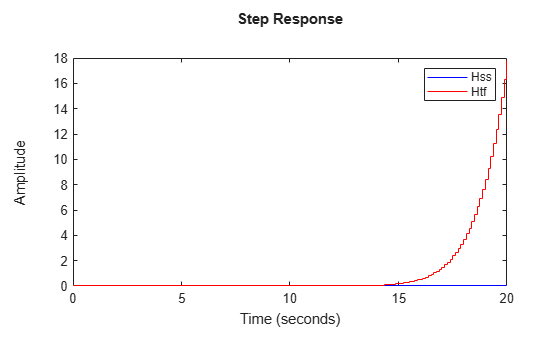The step response of `Htf` diverges even though the state-space model `Hss` is stable (all its poles lie in the unit circle). The Bode plot also shows a large discrepancy between the state-space and transfer function models:

```bode(Hss,'b',Htf,'r--') legend('Hss','Htf')```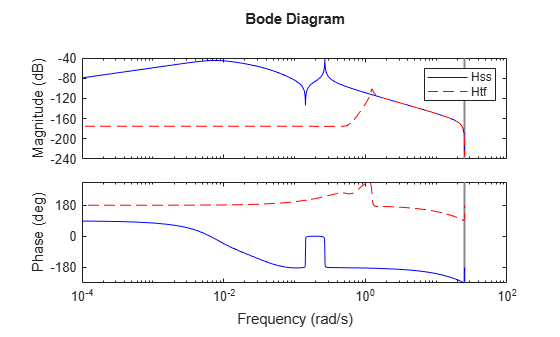The algorithm used to convert from state space to transfer function is not causing this discrepancy. If you convert from state space to zero-pole-gain, the first step in any SS to TF conversion, the discrepancies disappear:

```Hzpk = zpk(Hss); step(Hss,'b',Hzpk,'r--') legend('Hss','Hzpk')```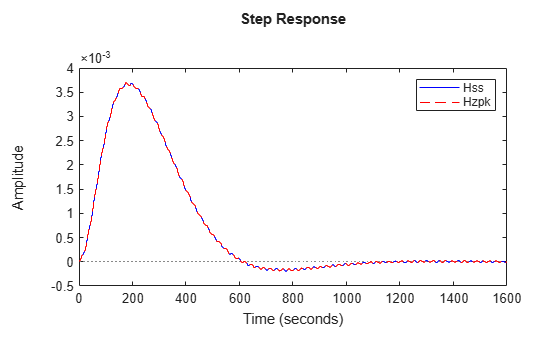`bode(Hss,'b',Hzpk,'r--')`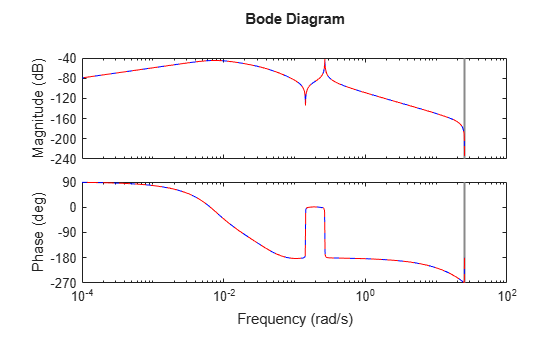This analysis shows that discrepancies arise in the ZPK to TF conversion, which merely involves computing a polynomial from its roots.

### Cause of Discrepancy

To understand the cause of these large discrepancies, compare the pole/zero maps of the state-space model and its transfer function:

```pzplot(Hss,'b',Htf,'r') legend('Hss','Htf')```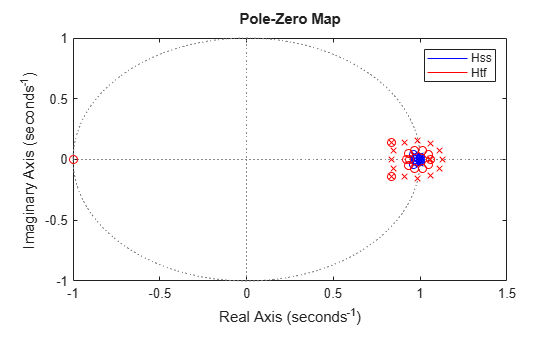Note the tightly packed cluster of poles near z=1 in `Hss`. When these poles are recombined into the transfer function denominator, roundoff errors perturb the pole cluster into an evenly-distributed ring of poles around z=1 (a typical pattern for perturbed multiple roots). Unfortunately, some perturbed poles cross the unit circle and make the transfer function unstable. Zoom in on the plot to see these poles:

```pzplot(Hss,'b',Htf,'r'); axis([0.5 1.5 -.4 .4])```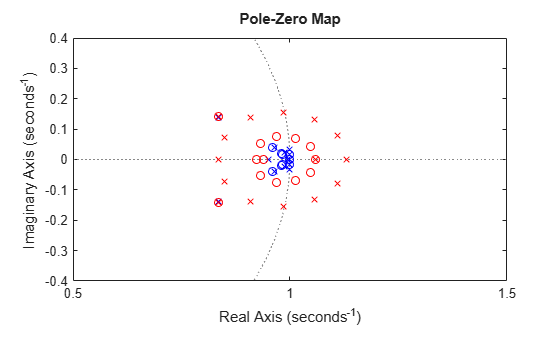You can confirm this explanation with a simple experiment. Construct a polynomial whose roots are the poles `R1` of `Hss`, compute the roots of this polynomial, and compare these roots with `R1`:

```R1 = pole(Hss); % poles of Hss Den = poly(R1); % polynomial with roots R1 R2 = roots(Den); % roots of this polynomial plot(real(R1),imag(R1),'bx',real(R2),imag(R2),'r*') legend('R1','roots(poly(R1))');```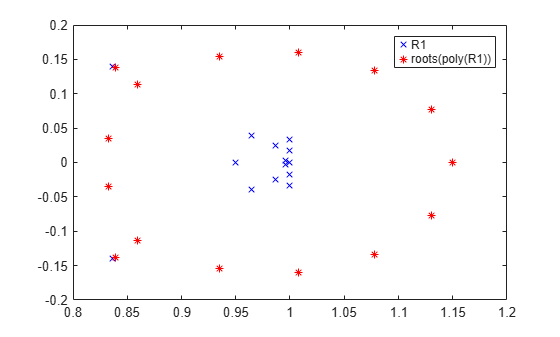This plot shows that `ROOTS(POLY(R1))` is quite different from `R1` because of the clustered roots. As a result, the roots of the transfer function denominator differ significantly from the poles of the original state-space model `Hss`.

In conclusion, you should avoid converting state-space or zero-pole-gain models to transfer function form because this process can incur significant loss of accuracy.

## Support

#### Learn how to automatically tune PID controller gains

Download code examples